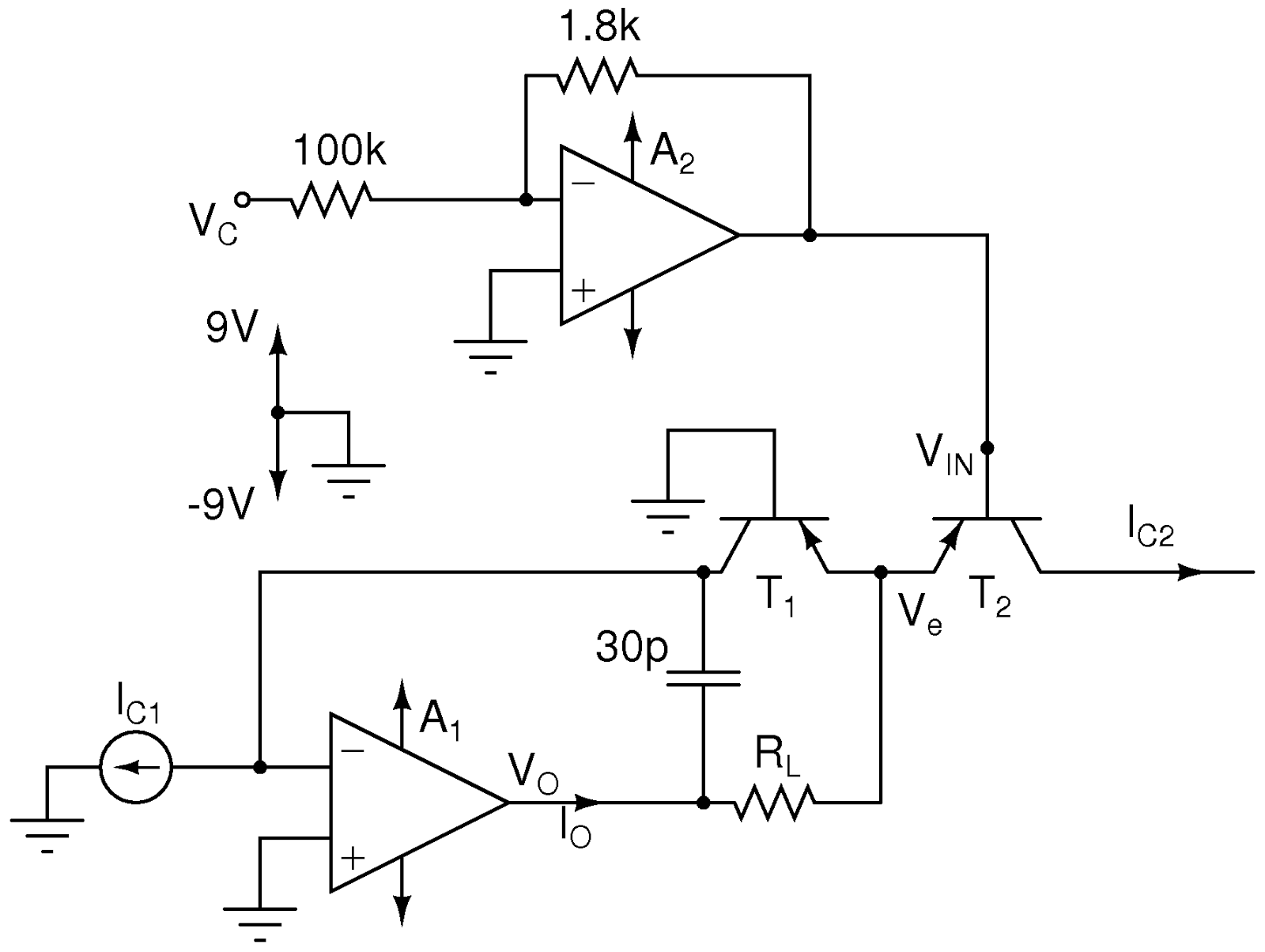## Thursday, January 9, 2014

### Logarithmic Converter, Control Voltage to Logarithmic Current, Part 1, theory

One standard of analogue synthesizer control is 1V/octave. This is what I'm implementing in the MIDI2VC, and now I need to build a simple logarithmic converter to place between the MIDI2VC and the VCO. In fact, I will actually build a new, really simple VCO from a LM13700.
The logarithmic converter is thoroughly explained in Electronotes S-019. The one described on page 2 is one that sinks current, and the LM13700 VCO needs a current source. In Electronotes 129 there is a big VCO schematic that has a log converter with a current source. A combination of the two schematics is seen here:Current source logarithmic converter
The relationship between the currents is:
$I_{C2 } = I_{C1} e^{-V_{IN}\cdot \ 26 \text{mV} }$
Ignoring the small base currents in the transistors, we also have
$I_{O } = I_{C1}+I_{C2}$
We will want to limit  $I_{C2}$ to protect the LM13700. It can receive a current of 2mA, but let's set max current to 1mA to be on the safe side. We can combine the two current equations to:
$I_{O } = I_{C2} ( 1 + e^{V_{IN} / 26 \text{mV} } )$
$A_2$ is an inverting attenuator that converts positive control voltage to negative in voltage:
$V_{IN} = V_C \cdot 18 \text{mV}$
$V_O$ will swing all the way to the positive rail (or close at least). $V_e$ is always close to 0.6mV (diode drop). We want the converter to work up to 5 Volts (max output of the MIDI2VC). This means we should set
$R_L \le \frac{9-0.6 \mathrm{V}}{1\mathrm{mA} (1+e^{-5 \cdot 18/26} ) } = 8144 \Omega$
In fact, we need to go a bit lower on $R_L$, due to the approximations in the calculation. I settled fro $R_L = 6.8$k\Omega.
We now set:
$I_{C1} \le 1\mathrm{mA} e^{-5 \cdot 18/26}$
We can get this current by connecting 330k\Omega to the negative rail.
An LTSPICE simulation of this circuit can give us a plot like this:Output current as function of voltage. Note that the current doubles between each 1V increment up to the current limitation. Simulation done in LTSPICE.

#### 1 comment:

1.Hello from berlin,

i have read that the vintage Vermona Synhtesizer needs a logarithmik vc/cv.
Do you know something about that, or is it a wrong komment?

Regards
Ralf